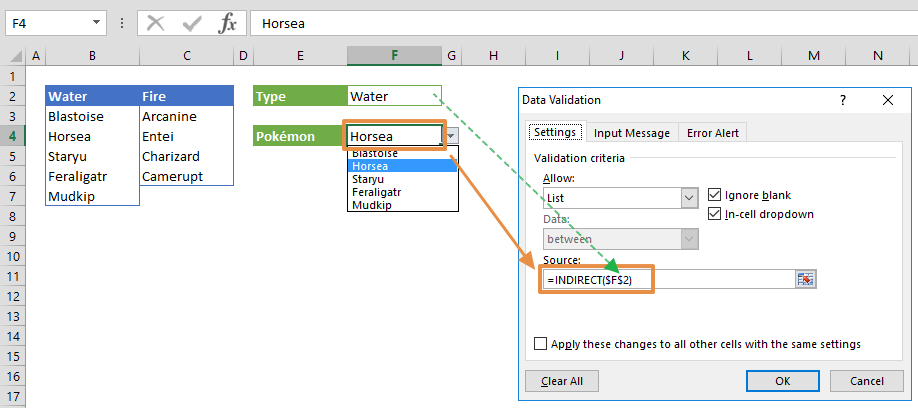The Excel INDIRECT function is a Lookup & Reference formula that returns the reference specified by a text string. It is useful for when you want to convert text strings into valid references. In this guide, we’re going to show you how to use the Excel INDIRECT function and also go over some tips and error handling methods.

# Supported versions

• All Excel versions

# Syntax

=INDIRECT(ref_text, [a1])

# Arguments

 ref_text A string value that represents a valid reference. This reference can be in A1 or R1C1 style. Specify [a1] argument for  R1C1 style. [a1] Optional. Specifies the reference type. TRUE: A1-style (Default) FALSE: R1C1-style

Examples

Note that we're using named ranges in our sample formulas to make them easier to read. This is not required.

## Single Cell

=INDIRECT("B3")

=INDIRECT("R[-2]C[-3]",FALSE)

formula is a simple use case for the INDIRECT function with both reference styles. Simply enter the references as strings with the corresponding ( [a1] ) argument.## Range and other functions

=COUNTA(INDIRECT("B"&Start_row&":B"&End_row))
formula is an example involving the Excel INDIRECT function where row values are retrieved from different references (Start_row, Send_row) and returned as a range for the COUNTA function. By setting Start_row 3 and End_row 14, we specify the range B3:B14, which contains 12 values.## External worksheets/workbooks

=INDIRECT("'"&Sheet_name&"'!"&Cell_ref)
formula uses the Excel INDIRECT function with a reference string that contains a syntax for an external worksheet. You can access the data from other worksheets or workbooks by entering a valid reference that points to an external source.

To create a valid external reference, make sure you follow these constraints:

• Cell/range reference should start with a "!" operator after workbook and/or worksheet name.
• Workbook name should be wrapped in brackets "[…]".
• If there is a space or special character in the workbook or worksheet name, the workbook-worksheet pair should be in single quotes ('…'). We recommend always using single quotes to avoid these types of issues.

A sample reference:

='[Pokémon Stats.xlsx]Generation I'!A2:G9## Dependent Dropdown

With dependent dropdowns, you can choose the ranges to be used in a list, and create a master list that contains these names. Using a name as ref_text argument of the INDIRECT function returns the range of the list.

For example, the cell F2 here has the value "Water", which is also the name of the range B3:B7. As a result, =INDIRECT(\$F\$2) formula returns the values in range B3:B7. If formula is applied as the Source of a Data Validation List, the values under the named range Water will be listed in the dropdown.See How to create dependent dropdowns for more details.